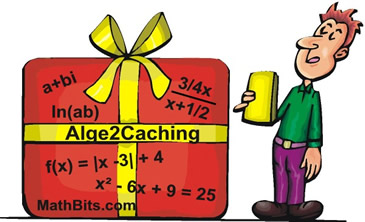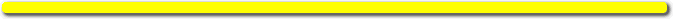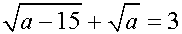MathBits.com presents ... .Welcome to the "Alge2Caching"
version of MathCaching!
(This level is for Algebra 2 students.)

Solve the following 3 problems:

 1. Which quadratic equation has roots of 5i and -5i? 1) x2 + 25 = 0 |2) x2 - 25 = 0 3) x2 + 10x + 25 = 0 4) x2 + 10x - 25 = 0

 2. Which of the numbered choices is the solution set to the equation x5 - 10x3 + 9x = 0? 1) {±1, ±3} 2) {0, ±1, ±3} 3) {±1, ±9} 4) {0, ±1, ±9}

 3. Which of the numbered choices is the solution to:1) 16 2) 4 3) -16 4) No solution